# How to Sum if Date is Greater Than A Date in Excel

In previous article, we have introduced you the method to sum if date is included in a period, you can check “How to Sum if Date Between Two Dates” on our website for reference. But sometimes we may want to sum numbers not for a period but just after a date. In this article, we will show you how to ‘sum if date is greater than a date’ by formula with the help of Excel SUMIF/SUMIFS function. We also introduce DATE function in case of date format is not a valid date format so that it cannot be used in a formula directly.

Through a simple instance, we will introduce you the syntax, arguments of SUMIF/SUMIFS function, and let you know how the formula works step by step to reach your goal finally. After reading the article, you may have a simple understanding of SUMIF/SUMIFS/DATE functions.

EXAMPLE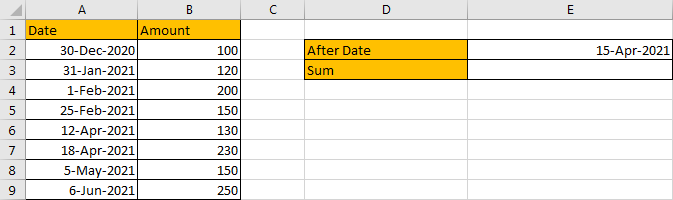Refer to above left-hand table, we can see that in the most left column “Date”, some dates are listed. And amount for each date is listed in “Amount” column accordingly.

In this instance, we want to sum numbers after a certain date. And this date is saved in “After Date” field in the right-hand table. This date is a dynamic date and we can change it properly based on our requirement. After the date is confirmed, only the dates which are later than the date can meet our condition. We need to find out all proper dates, and then sum up values in “Amount” column. To resolve this issue by formula, we can apply SUMIF or SUMIFS.

FORMULA – SUMIF FUNCTION

Step 1: Select A2:A9, then in Name Box define a new name for this range, for example ‘Date’.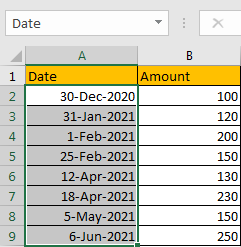Step 2: Select B2:B9, in Name Box define a new name for this range, for example ‘Amount’.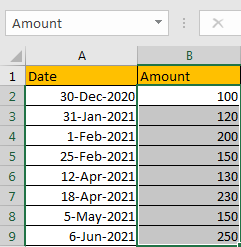Step 3: In E2, enter the formula =SUMIF(Date,”>”&DATE(2021,4,15),Amount).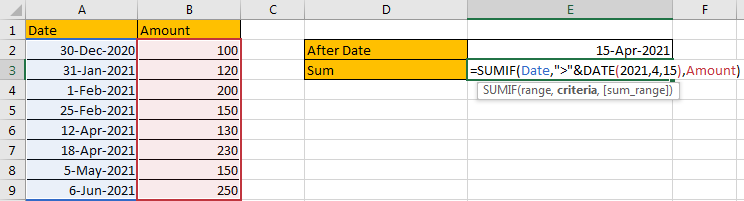NOTE: In step#1 and step#2 we defined range name “Date” and “Amount”, when entering the formula, after typing “Amou…”, defined range “Amount” is auto loaded, you can directly select it from dropdown list. You can also select B2:B9, A2:A9 to fill formula arguments as well. Be aware that don’t select DATE function in the dropdown list.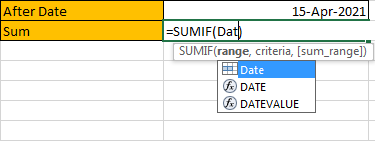Step 4: Press Enter after typing the formula.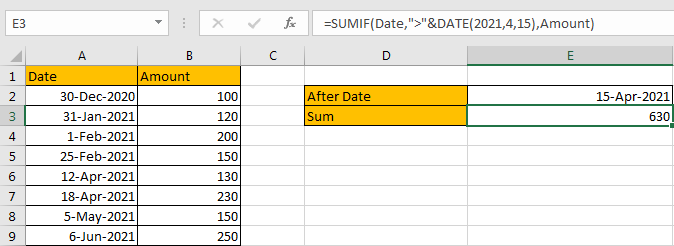We can see in column A, cell A7 (4/18/2021), A8 (5/5/2021) and A9 (6/6/2021) are after date “4/15/2021”, so the corresponding amounts are 230 (in cell B7), 150 (in cell B8), and 250 (in cell B9), so the total amount is 230+150+250=630. The formula works correctly.

FORMULA – SUMIFS FUNCTION

We can also apply SUMIFS function to resolve this issue.

Step 1: In E2, enter the formula =SUMIFS(Amount,Date,”>”&DATE(2021,4,15)).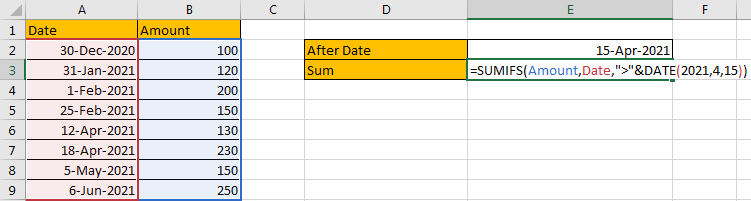Step 2: Press Enter after typing the formula.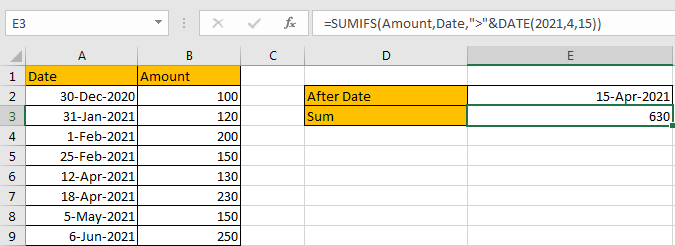We can see that the difference between SUMIF and SUMIFS is argument order. Compare with SUMIF, SUMIFS function list sum range at first, then criteria range, and criteria lists in the end. Besides, SUMIFS function allows multiple “criteria range and criteria” combinations.

FUNCTION INTRODUCTION

1. SUMIF Function

SUMIFS function can be seen as SUM+IF, it can sum based on criteria.

For SUMIF function, the syntax is:

=SUMIF (range, criteria, [sum_range]) – Argument in [] is optional. If sum range is omitted, SUMIF will sum up all numbers in range argument.

2. SUMIFS Function

SUMIFS function can be seen as SUM+IFS, it can handle multiple ‘criteria range’ and ‘criteria’ combinations.

For SUMIFS function, the syntax is:

SUMIFS(sum_range, criteria_range1, criteria1, [criteria_range2, criteria2], …). Contents in [] are optional.

Both SUMIF and SUMIFS function allow wildcards like asterisk ‘*’ and question mark ‘?’, they also allow logical operators within arguments. If wildcards or logical operators are required, they should be enclosed into double quotes (““) with text.

The usage of wildcards:

1. An asterisk (*) means one or more characters.
2. A question mark (?) means one character.
3. The position of asterisk or question mark means the character(s) position relative to the entered part. For example, “*A*” means characters or texts are both listed before and after “A”. “A*” means this text is started with A, but ends with others.

The usage of logical operators:

1. “>” – greater than
2. “>=” – greater than or equal to
3. “<” – less than
4. “<” – less than or equal to
5. “<>” – not equal to
6. To connect logical operator and text, we need to add an ampersand “&” to concentrate them. For example “>” & 100 or “<=” & E2.
7. DATE Function

DATE function can return a serial number that can represent a particular date if current cell format is ‘General’ or directly return a date if current cell format is ‘Date’. The syntax is

DATE(year,month,day) – All the three arguments ‘year’,’month’,’day’ are required.

ARGUMENTS

Let’s introduce the arguments of used functions.

DATE – YEAR, MONTH, DAY

If date needs to be hard coded in a formula, we often use DATE function. In this case, date is 15-Apr-2021, so year=2021, month=4, day=15.

In the formula bar, select ‘”>”&Date(2021,4,15)’, press F9, date is converted to a five digits number in the formula.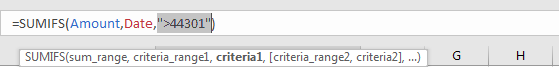In this case, SUMIF and SUMIFS have the same sum range, criteria range and criteria references, and working processes are similar, so we only clarify SUMIFS function in the following parts.

SUMIFS – SUM RANGE

In our instance, B2:B9 is the ‘sum range’. Amount values are listed in this field. We define this range with name ‘Amount’ in above step#2.

In the formula bar, select ‘Amount’, press F9, values in this range are listed in an array.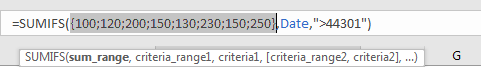SUMIFS – CRITERIA RANGE

A2:A9 is the criteria range. In this instance we have only one criteria range. This range contains different dates.

In the formula bar, select ‘Date’, press F9, values in this range are listed in an array.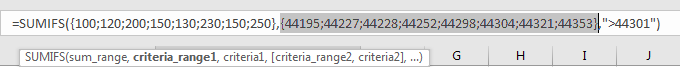In this step, dates are converted from Date format to General format, a five digits number is displayed to represent a date. Date 12/30/2020 in A2 is the earliest date in the list, so its relative five digits number 44195 is the smallest in the array, and date 6/6/2021 is the latest date in the list, so 44353 is the largest among all numbers in the array.

SUMIFS – CRITERIA

We want to calculate total amount after date “4/15/2021”. As in the table ‘’ is not a valid date, so we cannot directly use cell reference in the formula, so DATE function is applied here. As the target date should be after “4/15/2021”, so “>” is added. The complete criteria is “>”&DATE(2021,4,15).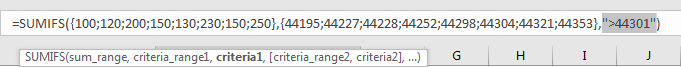HOW FORMULA WORKS

After explaining each argument in the formula, now we will show you how the formula works with these arguments.

Refer to above mentioned arguments, the formula is converted into below format in the formula bar.

=SUMIFS({100;120;200;150;130;230;150;250},{44195;44227;44228;44252;44298;44304;44321;44353},”>44301″)

We have below pair of criteria range and criteria:

Criteria range: {44195;44227;44228;44252;44298;44304;44321;44353}

Criteria: “>44301”

In criteria range, compare each number in the array with criteria 44301; if number can satisfy the condition “>44301”, mark it bold in array:

{44195;44227;44228;44252;44298;44304;44321;44353} – Bold if it is >44301

Now, for bold numbers, they can meet our requirement, so record a ‘True’ for them in the array; otherwise, record a ‘False’ for others. Then we can get a new array:

{Flase;False;False;False;False;True;True;True}

Convert ‘True’ to ‘1’ and ‘False’ to ‘0’ to apply this array into calculation in the following steps:

{0;0;0;0;0;1;1;1}

Now, we have below two arrays:

{100;120;200;150;130;230;150;250} – Sum Range

{0;0;0;0;0;1;1;1} – from above steps we know that if date is after “4/15/2021”, 1 is displayed.

We list the two arrays in two rows, in the same column multiply the two numbers, and save their product in another row.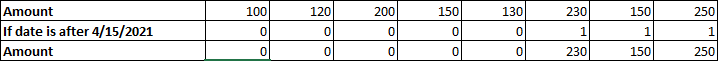Sum up all numbers in the third row.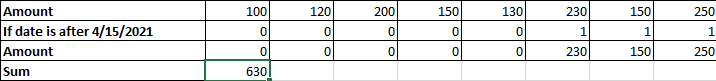230+150+250=630

COMMENT

1.If “After Date” value is changed in E2, we need to update DATE function year, month, date values manually.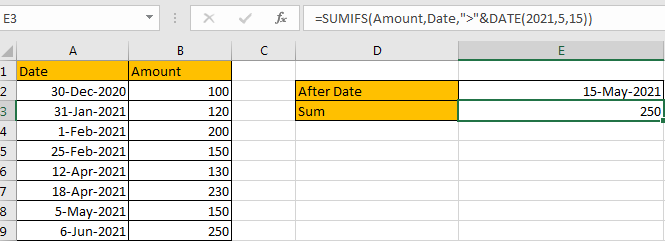2.If date is a valid date like 4/15/2021, we can directly use cell reference in the formula. See example below.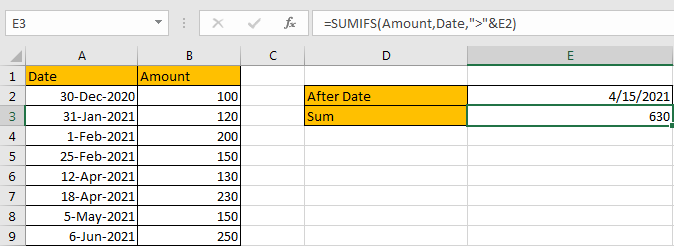SUMMARY

1. SUMIFS function can handle multiple pairs of criteria ranges and criteria. Sum range is the first argument among all arguments.

2. SUMIF function can handle one pair of criteria range and criteria. Sum range is the last argument among all arguments.

3. They allow user defined range name, wildcards, logical operators.

If you want to hard-code date in formula, you can apply DATE function.

### Related Functions

• Excel SUMIFS Function
The Excel SUMIFS function sum the numbers in the range of cells that meet a single or multiple criteria that you specify. The syntax of the SUMIFS function is as below:=SUMIFS (sum_range, criteria_range1, criteria1, [criteria_range2, criteria2], …)…
• Excel DATE function
The Excel DATE function returns the serial number for a date.The syntax of the DATE function is as below:= DATE (year, month, day)…
• Excel SUMIF Function
The Excel SUMIF function sum the numbers in the range of cells that meet a single criteria that you specify. The syntax of the SUMIF function is as below:=SUMIF (range, criteria, [sum_range])…